### Proportional Rates/Means Models for Recurrent Events

Let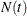be the number of events experienced by a subject over the time interval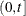. Let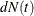be the increment of the counting process N over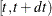. The rate function is given by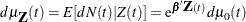where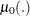is an unknown continuous function. If theare time independent, the rate model is reduced to the mean model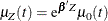The partial likelihood for n independent triplets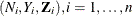, of counting, at-risk, and covariate processes is the same as that of the multiplicative hazards model. However, a robust sandwich estimate is used for the covariance matrix of the parameter estimator instead of the model-based estimate.

Let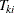be the kth event time of the ith subject. Let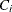be the censoring time of the ith subject. The at-risk indicator and the failure indicator are, respectively,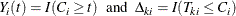Denote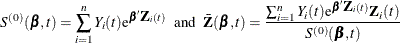Let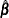be the maximum likelihood estimate of, and let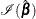be the observed information matrix. The robust sandwich covariance matrix estimate is given by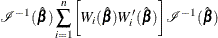where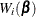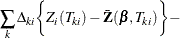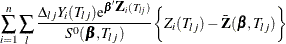For a given realization of the covariates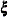, the Nelson estimator is used to predict the mean function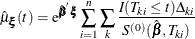with standard error estimate given by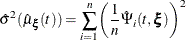where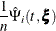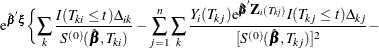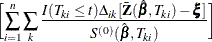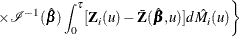Since the cumulative mean function is always nonnegative, the log transform is used to compute confidence intervals. The% pointwise confidence limits for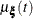are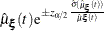where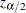is the upper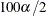percentage point of the standard normal distribution.